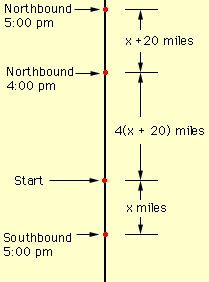Name: amy Who is asking: Student Level: Middle Question: a north bound train left at noon. four hours later a south bound train left the same station. at 5 o'clook they were 160 miles apart. what was the rate of both if the north bound train was going 20 miles faster than the other one. clark's final exam was given 5 times the weight of the weight of a weekly test. the avgerage of 15 weekly test was 91. the overall weighted average was 85. what did he make on the final. solve: -c-3(c-4)-9^0(c-2)=-6(c-4) x^2+6 -6/x^3 -9x^2+20x / x^2+x-6/x^3-2x^2-15x divide: -2x^3+1 by x-1 Hi Amy, Here is some help on a couple of your problems. Problem 1. Draw a diagram. Let x mph be the speed of the southbound train then the speed of the northbound train is x + 20 mph. In the first 4 hours after noon the northbound train goes 4(x + 20) miles. In the next hour it goes a further x + 20 miles and the southbound train goes x miles. From the diagram the distance between the trains at 5:00 pm is (x + 20) + 4(x + 20) + x miles. Set this equal to 160 miles and solve for x.Problem 2 Suppose that the sum of Clark's scores on the 15 weekly tests is x. If the weight of each of the 15 weekly tests is 1 and the average is 91 then x/15 = 91. Thus x = 15(91) = 1365. If his score on the final exam is F, its weight is 5 and the overall weighted average is the sum of the weighted scores divided by the sum of the weights. That is (1365 + 5F)/(15 + 5). But this is 85 so solve (1365 + 5F)/(15 + 5) = 85 for F. Cheers, Penny Go to Math Central